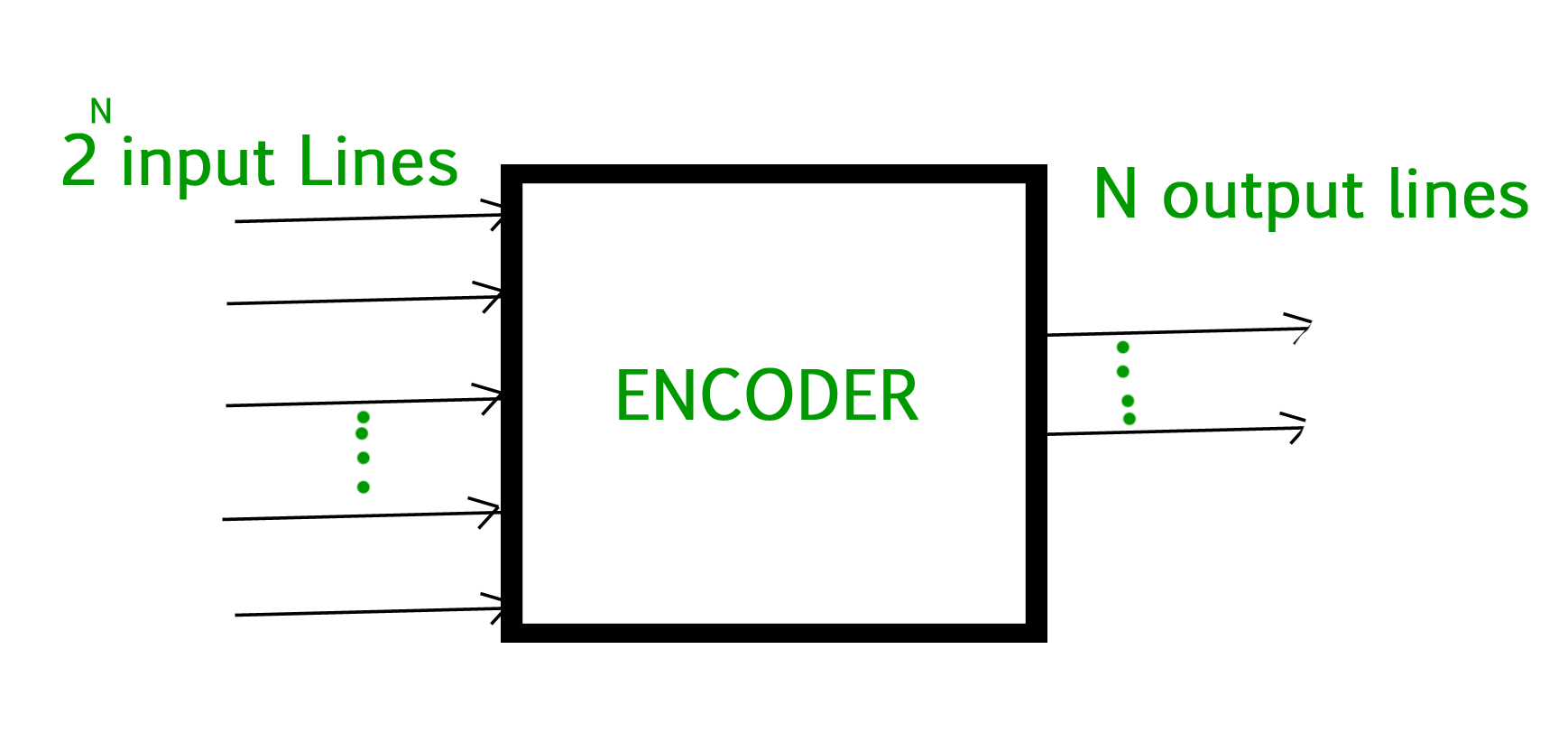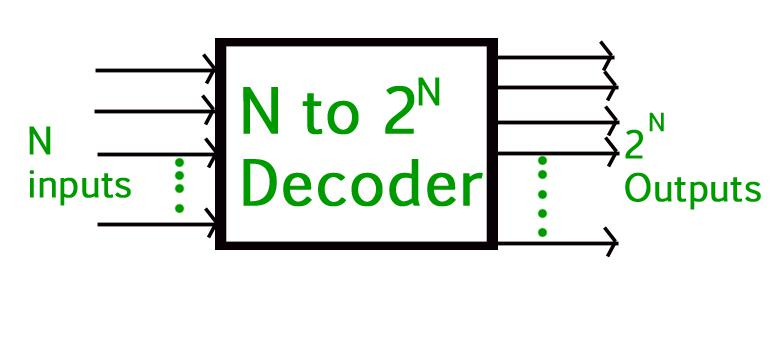# Difference Between Encoder and Decoder

Combinational Logic is the concept in which two or more input states define one or more output states. The Encoder and Decoder are combinational logic circuits. In which we implement combinational logic with the help of boolean algebra.

To encode something is to convert an unambiguous piece of information into a form of code that is not so clearly understood and the device which performs this operation is termed ad Encoder.

## Encoder

An Encoder is a device that converts the active data signal into a coded message format or it is a device that converts analogue signal to digital signals. It is a combinational circuit, that converts binary information in the form of 2N input lines into N output lines which represent N bit code for the input. When an input signal is applied to an encoder the logic circuitry involved within it converts that particular input into coded binary output.To decode is to perform the reverse operation: converting a code back into an unambiguous form code and the device which performs this operation is termed a Decoder.

## Decoder

A decoder is also a combinational circuit as an encoder but its operation is exactly reverse as that of the encoder. A decoder is a device that generates the original signal as output from the coded input signal and converts n lines of input into 2n lines of output. An AND gate can be used as the basic decoding element because it produces a high output only when all inputs are high.Decoder

## Encoder vs Decoder

ENCODER

DECODER

Encoder circuit basically converts the applied information signal into a coded digital bit stream.

Decoder performs reverse operation and recovers the original information signal from the coded bits.

In case of encoder, the applied signal is the active signal input.

Decoder accepts coded binary data as its input.

The number of inputs accepted by an encoder is 2n.

The number of input accepted by decoder is only n inputs.

The output lines for an encoder is n.

The output lines of an decoder is 2n.

The encoder generates coded data bits as its output.

The decoder generates an active output signal in response to the coded data bits.

The operation performed is simple.

The operation performed is complex.

The encoder circuit is installed at the transmitting end.

The decoder circuit is installed at the receiving side.

OR gate is the basic logic element used in it.

AND gate along with NOT gate is the basic logic element used in it.

It is used in E-mail, video encoders etc.

It is used in Microprocessors, memory chips etc.